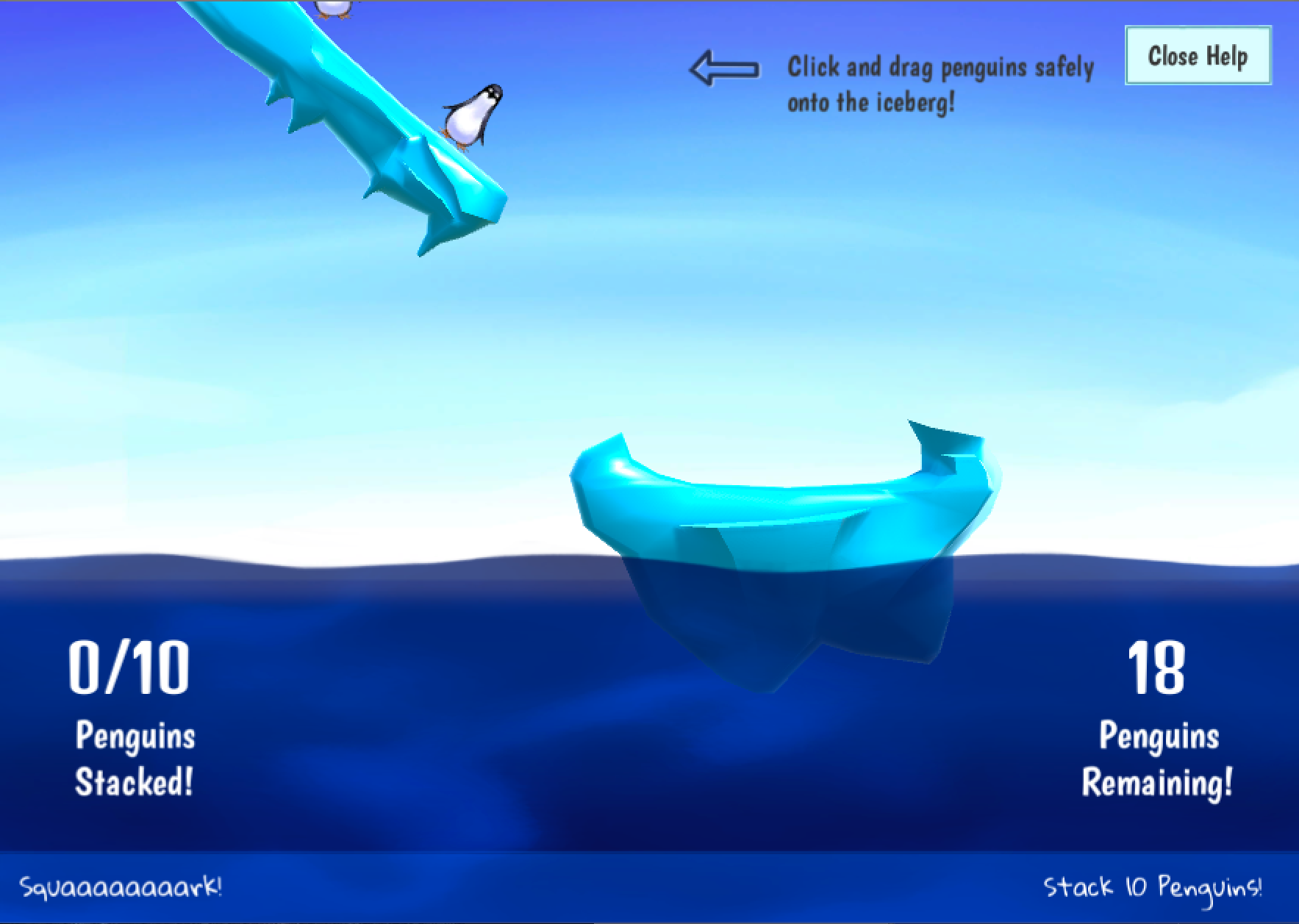# Penguins

So, my new goal for 2016 is to make (and finish, and publish!) one little game per month for the rest of the year.

They don't have to anything amazing, but the idea is that they do actually have to be done and finished at the end.

I do write a lot of bits and pieces, but the reason this blog hardly ever gets updates is that I never finish things, and that's gonna change now.The lovely risachantag kindly did all the arts for this one and helped heaps with the level building.

There's a WebGL build of it up here! (14MB!)

It probably won't run well (or at all) on phones; I recommend a tablet or desktop browser. :)

For future games I might put up some random technical notes, but there's not much to say for this one except the 2D buoyancy effects are actually realllly tricky to work with.

Turns out that (obviously, once you think about it), the weight of an object doesn't control if it floats or not. Rather, its the weight vs. the volume of liquid is displaces. That's awesome, but it makes tweaking the settings pretty complicated when you're trying to adjust the center of mass for objects.

On a more positive note, it turns out forking the existing sprite shader to have a 'sprite' shader for the dynamic water was pretty easy.

I won't go into the details, but here's the one we ended up with:

``````Shader "Shaders/Sprites/CustomDefault"
{
Properties
{
[PerRendererData] _MainTex ("Sprite Texture", 2D) = "white" {}
_Offset ("Offset (RGB)", 2D) = "white" {}
_Mask ("Offset (RGB)", 2D) = "white" {}
_Amount ("Offset Amount", Range(0,1)) = 0.5
_MaskYAmount ("Mask Y Amount", Range(0,1)) = 0.5
_Speed ("Offset Speed", Range(0,1)) = 0.5
_Color ("Tint", Color) = (1,1,1,1)
[MaterialToggle] PixelSnap ("Pixel snap", Float) = 0
}

{
Tags
{
"Queue"="Transparent"
"IgnoreProjector"="True"
"RenderType"="Transparent"
"PreviewType"="Plane"
"CanUseSpriteAtlas"="True"
}

Cull Off
Lighting Off
ZWrite Off
Blend One OneMinusSrcAlpha

Pass
{
CGPROGRAM
#pragma vertex vert
#pragma fragment frag
#pragma multi_compile _ PIXELSNAP_ON
#include "UnityCG.cginc"

#define IF(a, b, c) lerp(b, c, step((fixed) (a), 0))

uniform float _Amount;
uniform float _Speed;

struct appdata_t
{
float4 vertex   : POSITION;
float4 color    : COLOR;
float2 texcoord : TEXCOORD0;
float2 tex2  : TEXCOORD1;
};

struct v2f
{
float4 vertex   : SV_POSITION;
fixed4 color    : COLOR;
float2 texcoord  : TEXCOORD0;
float2 tex2  : TEXCOORD1;
};

fixed4 _Color;

v2f vert(appdata_t IN)
{
v2f OUT;
OUT.vertex = mul(UNITY_MATRIX_MVP, IN.vertex);
OUT.texcoord = IN.texcoord;
OUT.tex2 = IN.tex2;
OUT.color = IN.color * _Color;
#ifdef PIXELSNAP_ON
OUT.vertex = UnityPixelSnap (OUT.vertex);
#endif

return OUT;
}

sampler2D _MainTex;
sampler2D _AlphaTex;
sampler2D _Offset;

fixed4 SampleSpriteTexture (float2 uv, float2 uv2)
{
// Sample the offset texture
fixed4 offset = tex2D (_Offset, uv2);

// Rotate the uv offset in a circle over the target texture
float v = _Amount * offset;
float x = uv + v * lerp(-1.0, 1.0, sin(_Time * _Speed));
float y = uv + v * lerp(-1.0, 1.0, cos(_Time * _Speed));

// Wrap x & y
x = IF(x < 0, 1.0 - x, IF(x > 1, x - 1.0, x));
y = IF(y < 0, 1.0 - y, IF(y > 1, y - 1.0, y));

// No y wrap for mask
float my = clamp(uv + _MaskYAmount * v * lerp(-1, 1, abs(cos(_Time * _Speed))), 0, 0.9);
float mx = uv + _Time * _Speed;
fixed4 mask = tex2D (_Mask, float2(mx, my));

// Get color
fixed4 color = tex2D (_MainTex, float2(x, y));
#if ETC1_EXTERNAL_ALPHA
// get the color from an external texture (usecase: Alpha support for ETC1 on android)
color.a = tex2D (_AlphaTex, uv).r;
#endif //ETC1_EXTERNAL_ALPHA

// Apply alpha mask
return color;
}

fixed4 frag(v2f IN) : SV_Target
{
fixed4 c = SampleSpriteTexture (IN.texcoord, IN.tex2) * IN.color;
c.rgb *= c.a;
return c;
}
ENDCG
}
}
}
``````

...and finally, although this was my 'April' game, it took the first two weeks of May to get it done.

Possibly a tiny bit ambitious for a first 'game per month' game, but hey.

Just means May is going to be... a little simpler. :)